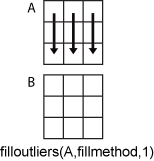# filloutliers

## 语法

``B = filloutliers(A,fillmethod)``
``B = filloutliers(A,fillmethod,findmethod)``
``B = filloutliers(A,fillmethod,'percentiles',threshold)``
``B = filloutliers(A,fillmethod,movmethod,window)``
``B = filloutliers(___,dim)``
``B = filloutliers(___,Name,Value)``
``````[B,TF,L,U,C] = filloutliers(___)``````

## 说明

``B = filloutliers(A,fillmethod)` 查找 `A` 中的离群值并根据 `fillmethod` 替换它们。例如，`filloutliers(A,'previous')` 将离群值替换为上一个非离群值元素。默认情况下，离群值是指与中位数相差超过三倍经过换算的中位数绝对偏差 (MAD) 的值。如果 `A` 是矩阵或表，则 `filloutliers` 分别对每一列进行运算。如果 `A` 是多维数组，则 `filloutliers` 沿大小不等于 1 的第一个维度进行运算。`

``B = filloutliers(A,fillmethod,findmethod)` 指定检测离群值的方法。例如，`filloutliers(A,'previous','mean')` 将 `A` 中与均值相差超过三倍标准差的元素定义为离群值。`
``B = filloutliers(A,fillmethod,'percentiles',threshold)` 将离群值定义为 `threshold` 所指定的百分位数以外的点。`threshold` 参数是包含上下百分位数阈值的二元素行向量，例如 `[10 90]`。`

``B = filloutliers(A,fillmethod,movmethod,window)` 指定根据 `window` 定义的窗口长度检测局部离群值的移动方法。例如，`filloutliers(A,'previous','movmean',5)` 将包含五个元素的窗口中与局部均值相差超过三倍局部标准差的元素标识为离群值。`

`对于上述任何语法，`B = filloutliers(___,dim)` 将沿 `A` 的维度 `dim` 运算。例如，`filloutliers(A,'linear',2)` 沿矩阵 `A` 的每一行运算。`

``B = filloutliers(___,Name,Value)` 使用一个或多个名称-值对组参数指定用来检测和替换离群值的其他参数。例如，`filloutliers(A,'previous','SamplePoints',t)` 相对于时间向量 `t` 中的对应元素检测 `A` 中的离群值。`

``````[B,TF,L,U,C] = filloutliers(___)``` 还返回检测方法计算的离群值和阈值的位置信息。`TF` 是一个逻辑数组，指示 `A` 中的离群值的位置。`L`、`U` 和 `C` 参数表示离群值检测方法使用的下阈值、上阈值和中心值。```

## 示例

```A = [57 59 60 100 59 58 57 58 300 61 62 60 62 58 57]; B = filloutliers(A,'linear'); plot(1:15,A,1:15,B,'o') legend('Original Data','Interpolated Data')``````A = [57 59 60 100 59 58 57 58 300 61 62 60 62 58 57]; B = filloutliers(A,'nearest','mean'); plot(1:15,A,1:15,B,'o') legend('Original Data','Interpolated Data')``````x = -2*pi:0.1:2*pi; A = sin(x); A(47) = 0;```

`t = datetime(2017,1,1,0,0,0) + hours(0:length(x)-1);`

```[B,TF,U,L,C] = filloutliers(A,'clip','movmedian',hours(5),'SamplePoints',t); plot(t,A,t,B,'o') legend('Original Data','Filled Data')````L(TF)`
```ans = -0.8779 ```

`A = randn(5,5) + diag(1000*ones(1,5))`
```A = 5×5 103 × 1.0005 -0.0013 -0.0013 -0.0002 0.0007 0.0018 0.9996 0.0030 -0.0001 -0.0012 -0.0023 0.0003 1.0007 0.0015 0.0007 0.0009 0.0036 -0.0001 1.0014 0.0016 0.0003 0.0028 0.0007 0.0014 1.0005 ```

```[B,TF,lower,upper,center] = filloutliers(A,0,2); B```
```B = 5×5 0 -1.3077 -1.3499 -0.2050 0.6715 1.8339 0 3.0349 -0.1241 -1.2075 -2.2588 0.3426 0 1.4897 0.7172 0.8622 3.5784 -0.0631 0 1.6302 0.3188 2.7694 0.7147 1.4172 0 ```

`[A(TF) B(TF)]`
```ans = 5×2 103 × 1.0005 0 0.9996 0 1.0007 0 1.0014 0 1.0005 0 ```

```x = 1:10; A = [60 59 49 49 58 100 61 57 48 58]; [B,TF,lower,upper,center] = filloutliers(A,'clip'); plot(x,A,x,B,'o',x,lower*ones(1,10),x,upper*ones(1,10),x,center*ones(1,10)) legend('Original Data','Filled Data','Lower Threshold','Upper Threshold','Center Value')```## 输入参数

`'center'`使用由 `findmethod` 决定的中心值进行填充
`'clip'`对于比 `findmethod` 决定的下阈值还小的元素，用下阈值填充。对于比 `findmethod` 决定的上阈值还大的元素，用上阈值填充。
`'previous'`使用上一个非离群值进行填充
`'next'`使用下一个非离群值进行填充
`'nearest'`使用最接近的非离群值进行填充
`'linear'`使用相邻的非离群值的线性插值进行填充
`'spline'`使用分段三次样条插值进行填充
`'pchip'`使用保形分段三次样条插值进行填充
`'makima'`修正 Akima 三次 Hermite 插值（仅限数值、`duration``datetime` 数据类型）

`'median'`离群值定义为与中位数相差超过三倍换算 MAD 的元素。换算 MAD 定义为 `c*median(abs(A-median(A)))`，其中 `c=-1/(sqrt(2)*erfcinv(3/2))`
`'mean'`离群值定义为与均值相差超过三倍标准差的元素。此方法比 `'median'` 快，但没有它可靠。
`'quartiles'`离群值定义为比上四分位数 (75%) 大 1.5 个四分位差以上或比下四分位数 (25%) 小 1.5 个四分位差以上的元素。当 `A` 中的数据不是正态分布时，此方法很有用。
`'grubbs'`使用 Grubbs 检验检测离群值，并基于假设检验每次迭代删除一个离群值。此方法假设 `A` 中的数据呈正态分布。
`'gesd'`使用广义极端 Student 化偏差检验检测离群值。此迭代方法与 `'grubbs'` 类似，但当有多个离群值互相遮盖时，此方法的执行效果更好。

`'movmedian'`离群值定义为在 `window` 指定的窗口长度内，与局部中位数相差超过三倍局部换算 MAD 的元素。
`'movmean'`离群值定义为在 `window` 指定的窗口长度内，与局部均值相差超过三倍局部标准差的元素。

`A` 是时间表或者 `'SamplePoints'` 被指定为 `datetime``duration` 向量时，`window` 的类型必须是 `duration`，而且将会基于样本点来计算窗口。

`filloutliers(A,fillmethod,1)` 根据每一列中的数据填充离群值。`filloutliers(A,fillmethod,2)` 根据每一行中的数据填充离群值。`A` 为表或时间表时，不支持 `dim``filloutliers` 分别对每个表或时间表变量进行运算。

### 名称-值对组参数

• 指定单个表变量名称的字符向量

• 字符向量元胞数组，其中每个元素都是表变量名称

• 表变量索引向量

• 逻辑向量，每个元素分别对应一个表变量，其中 `true` 指示包括对应变量，`false` 指示不包括对应变量。

• 以表为输入并返回逻辑标量的函数句柄

`'MaxNumOutliers'` 的默认值是最接近 `A` 中的元素数的 10% 的整数。为离群值数上限设置较大的值可以确保检测到所有离群值，但代价是降低计算效率。

## 详细信息

### 中位数绝对偏差

，其中 i = 1,2,...,N。